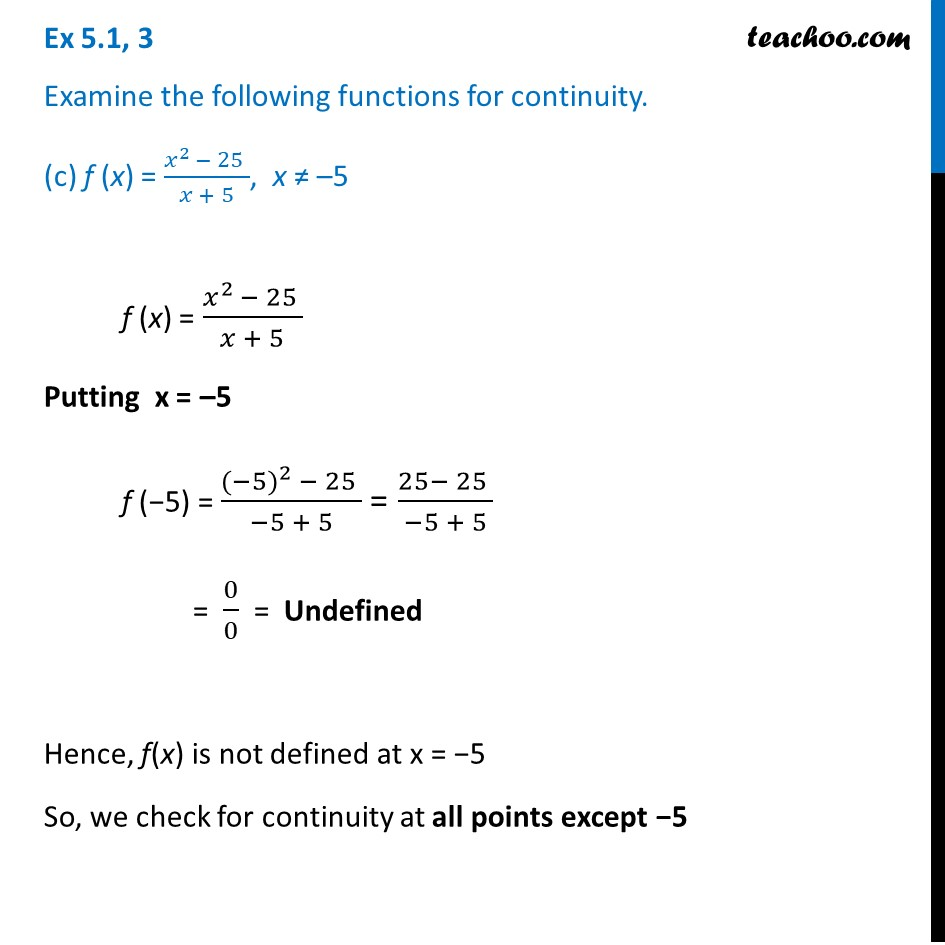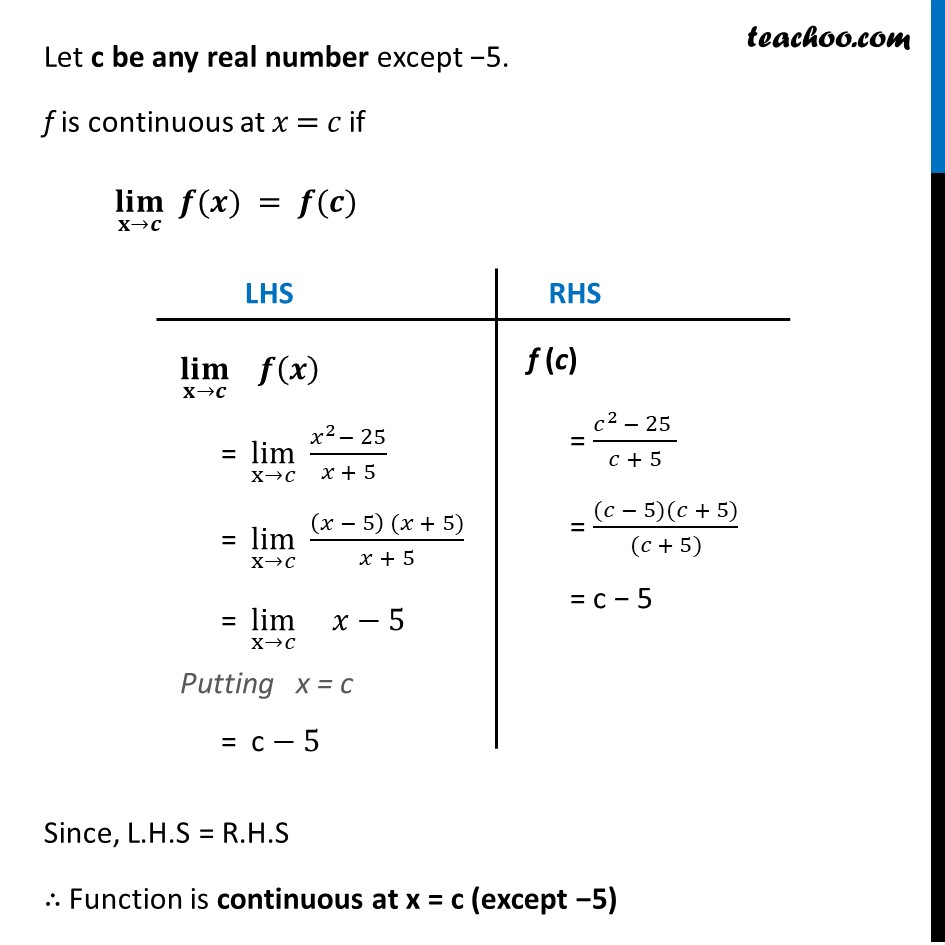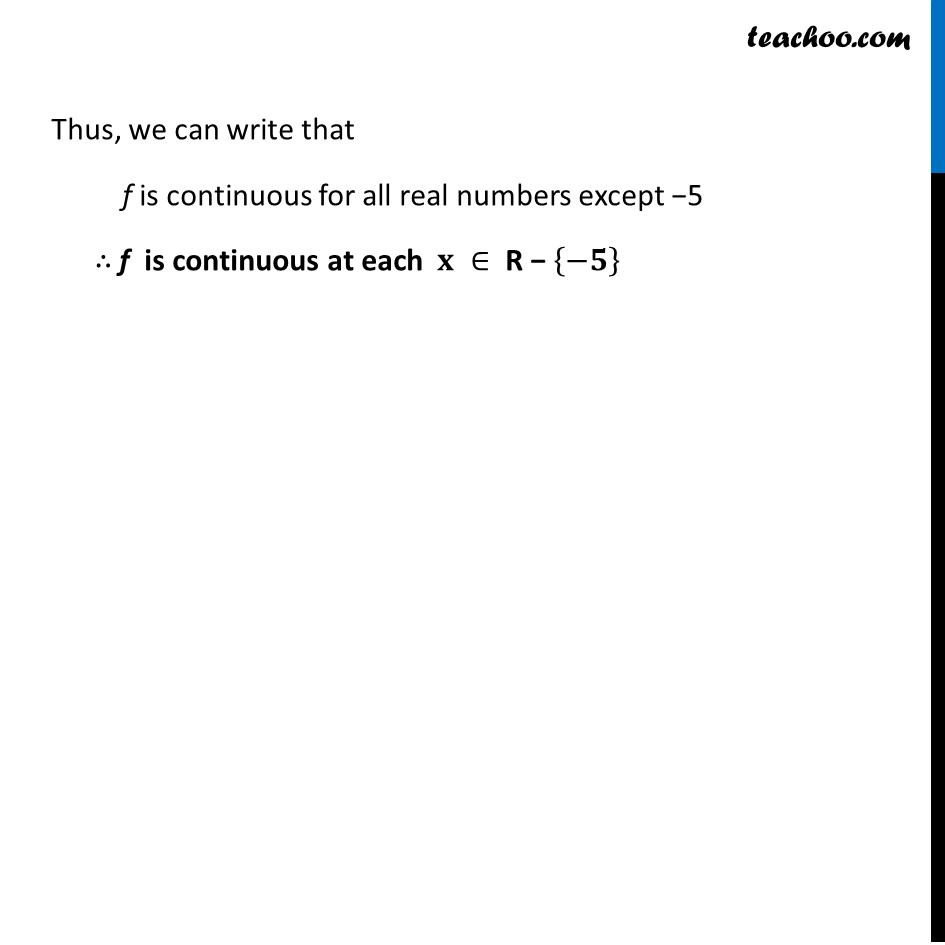Ex 5.1

Chapter 5 Class 12 Continuity and Differentiability
Serial order wiseLearn in your speed, with individual attention - Teachoo Maths 1-on-1 Class

### Transcript

Ex 5.1, 3 Examine the following functions for continuity. (c) f (x) = (𝑥^(2 )− 25 )/(𝑥 + 5), x ≠ –5 f (x) = (𝑥^(2 )− 25 )/(𝑥 + 5) Putting x = –5 f (−5) = (〖(−5)〗^(2 )− 25 )/(−5 + 5) = (25− 25 )/(−5 + 5) = 0/0 = Undefined Hence, f(x) is not defined at x = −5 So, we check for continuity at all points except −5 Let c be any real number except −5. f is continuous at 𝑥 = 𝑐 if (𝐥𝐢𝐦)┬(𝐱→𝒄) 𝒇(𝒙) = 𝒇(𝒄) LHS (𝐥𝐢𝐦)┬(𝐱→𝒄) 𝒇(𝒙) = lim┬(x→𝑐) (𝑥^2− 25)/(𝑥 + 5) = lim┬(x→𝑐) ((𝑥 − 5) (𝑥 + 5))/(𝑥 + 5) = lim┬(x→𝑐) 𝑥−5 Putting x = c = c − 5 RHS f (c) = (𝑐^(2 )− 25 )/(𝑐 + 5) = ((𝑐 − 5)(𝑐 + 5))/((𝑐 + 5)) = c − 5 Let c be any real number except −5. f is continuous at 𝑥 = 𝑐 if (𝐥𝐢𝐦)┬(𝐱→𝒄) 𝒇(𝒙) = 𝒇(𝒄) Since, L.H.S = R.H.S ∴ Function is continuous at x = c (except −5) Thus, we can write that f is continuous for all real numbers except −5 ∴ f is continuous at each 𝐱 ∈ R − {−𝟓}